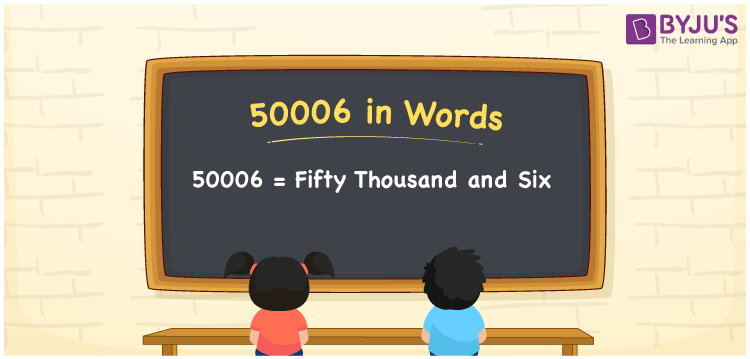# 50006 in words

50006 in words is written as Fifty Thousand and Six. In 50006, 5 has a place value of ten thousand and 6 is in the place value of one. The article on Place Value gives more information. The number 50006 is used in expressions that relate to money, distance, length, social media views, and many more. For example, “My last weekend’s shopping bill was Fifty Thousand and Six rupees, out of which six rupees was charged for a paper bag”.

 50006 in words Fifty Thousand and Six Fifty Thousand and Six in Numbers 50006

## 50006 in English Words## How to Write 50006 in Words?

We can convert 50006 to words using a place value chart. This can be done as follows. The number 50006 has 5 digits, so let’s make a chart that shows the place value up to 5 digits.

 Ten thousand Thousands Hundreds Tens Ones 5 0 0 0 6

Thus, we can write the expanded form as:

5 × Ten thousand + 0 × Thousand + 0 × Hundred + 0 × Ten + 6 × One

= 5 × 10000 + 0 × 1000 + 0 × 100 + 0 × 10 + 6 × 1

= 50006.

= Fifty Thousand and Six.

50006 is the natural number that is succeeded by 50005 and preceded by 50007.

50006 in words – Fifty Thousand and Six.

Is 50006 an odd number? – No.

Is 50006 an even number? – Yes.

Is 50006 a perfect square number? – No.

Is 50006 a perfect cube number? – No.

Is 50006 a prime number? – No.

Is 50006 a composite number? – Yes.

## Solved Example

1. Write the number 50006 in expanded form

Solution: 5 x 10000 + 0 x 1000 + 0 x 100 + 0 x 10 + 6 x 1

We can write 50006 = 50000 + 0000 + 000 + 00 + 6

= 5 x 10000 + 0 x 1000 + 0 x 100 + 0 x 10 + 6 x 1.

## Frequently Asked Questions on 50006 in words

Q1

### How to write the number 50006 in words?

50006 in words is written as Fifty Thousand and Six.
Q2

### Is 50006 divisible by 2?

Yes. 50006 is divisible by 2.
Q3

### Is 50006 a prime number?

No. 50006 is not a prime number.